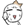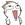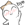# PHP基础语法之数组

### 数组的定义

<?php
/* 数组的定义 */

\$arr = array(1,2,3,4,5,6);      //创建一个整型数组，其值分别为1,2,3,4,5,6
echo "数组\\$arr的详细：<br>\n";
var_dump(\$arr);         //输出数组的详细信息
echo "打印\\$arr:<br>\n";
print_r(\$arr);          //可以看到默认键名为0开始依次继续

\$array_2 = array('a'=>'11','b'=>'22',"dsf","test");
echo "<br><br>数据\\$array_2的列表：<br>";
print_r(\$array_2);      //输出后看到序号分别为a,b,0,1,在于，函数会为每一个值分配一个序号

\$string_arr = array('a'=>'1','ff'=>'f','31'=>5,4,65,34);
echo "<br><br>\\$string_arr打印的列表：<br>\n";
print_r(\$string_arr);   //输出后可以看到，最还是得输出的是我们自己定义的键值，后面的从32开始排列,因为出现了一个前面有序号31
echo var_dump(\$string_arr);     //对数据信息进行详细输出，此处会发现数据的数据类型不是唯一的，会自动进行变换，这就是动态语言

\$array3 = ['a','b','c','a'=>'9','f'];           //这是数组的另一种定义方式，支持PHP5.4及以上，当然仅仅是将array函数去掉了而已
if(is_array(\$array3)){      //该函数可以判定是否为一个数组，还有类似的is_numeric判定是数字
echo "\\$array3是一个数组<br>\n";
print_r(\$array3);
echo "<br>当前类型为:".gettype(\$array3);       //还是函数可以输出变量的类型，然后进行相应的操作
}else{
echo "\\$array3不是一个数组，是一个".gettype(\$array3);
}

?>

### 数组的遍历

<?php
/* 数组的遍历 */

\$arr = array("a", "b", "c", "d", "e", 1, 2);
echo "打印数组列表:\n";
print_r(\$arr); //此时可以看到，该函数会将数组的详细信息打印出来
echo "使用for循环进行遍历：\n";
for (\$i = 0; \$i < count(\$arr); \$i++) {
//count函数会统计数组的个数
echo "第 \$i 个元素为:\$arr[\$i]\n";
//可以看到此时同样能将数组的元素写在字符串中，但往往这样会出错，这是一个特例。这里会有一个小bug就是，\$i不能和个连接在一起，否则会出错
}

echo "\n使用foreach遍历:\n";
foreach (\$arr as \$v) {
echo "元素值为:" . \$v, "\n"; //这里没有使用\$arr[?]的形式，在于每一次foreach都会将值赋给\$v
echo "ASCII码为:" . ord(\$v) . "\n"; //可以利用这个ord函数获取字符的ascii码,如果是数字，同样会转换为ascii码
}

\$lan = array("china" => "中国", "jan" => "日本", "usa" => "美国", "英国" => "u??忘了"); //这里可以看到，键名并非一定得为英文或数字，啥都行
echo "\n这样一个数组：\n";
print_r(\$lan);
echo "单独对一个进行输出:" . \$lan["china"]; //很显然这里不适合那种方式进行输出，这里键名中存在字符串，会引起语法错误
foreach (\$lan as \$id => \$val) { //此处使用了 => 该语法，表示将键名赋给\$id,值赋给\$val
echo "\$id=>\$val,且`\$val`的长度为" . strlen(\$val) . "\n";
//函数strlen(?)表示取得字符串的长度注意，如果将变量写在字符串中会有一定的小冲突，此时会需要使用空格等分割，或者使用连接符
//此时一个中文占3个长度，需要注意
}
?>

### 数组的修改

<?php
/* 数组的修改 */
\$arr = array("a","b","c");
echo "输出数组：\n";
print_r(\$arr);

echo "\n添加一个元素:\\$arr[] = rand();\n";
\$arr[] = rand();    //添加一个随机数，数组中键名留空表示使用默认键名
print_r(\$arr);

echo "\n修改第一个元素:\\$arr='my'\n";
\$arr = 'my';
print_r(\$arr);

echo "\n删除第0个元素:unset(\\$arr)\n";
unset(\$arr);     //删除后会发现元素值不改变
print_r(\$arr);

echo "\n自定义键值:\\$arr['name']='smith'\n";
\$arr['name']='smith';
print_r(\$arr);

echo "\n天机当前时间到数组:\n";
date_default_timezone_set('PRC');   //设置当前时区，如果不设置可能会产生一个警告，PRC表示中国
\$arr[] = date("Y-m-d H:i:s");       //产生一个时间，使用Data进行格式化
print_r(\$arr);  //打印后会发现该数的键值为4，因为之前删除的对值无影响

?>

### 数组的操作

<?php
/* 数组的操作 */

\$arr = array(); //创建一个空数组

\$n = rand(15,20);   //创建一个15,20的随机数
for(\$i = 0; \$i < \$n; \$i++)
{
\$x = rand()%50; //用一个随机数，并且取除以50的余数
if(isset(\$arr[\$x]) == false)    //判断\$arr[\$x]这个元素是否存在，如果存在返回true,否则返回false，此处可以用全等===
\$arr[\$x."a"] = rand();      //将随机数赋给当前元素
}
echo "原本要产生\$n 个随机数，但实际产生了".count(\$arr)."个元素\n";
print_r(\$arr);

echo "\n数组排序:sort(\\$arr)\n";
sort(\$arr);
print_r(\$arr);//此时键名会改变重置，但数组值不会改变

while(!empty(\$arr)){        //empty表示判断变量是否为空，如果元素为空则返回true,当多维数组时可能出错
\$v = array_pop(\$arr);       //该函数相当于出栈操作,永远选择的是最后一个元素
echo "\$v 被弹出\n";
}
echo "\n输出弹出元素的数组：";
print_r(\$arr);

echo "\n\\$arr数组进行一个元素的进栈操作\n";
array_push(\$arr,"test");
print_r(\$arr);

array_push(\$arr,"a","b");//将两个元素进栈
print_r(\$arr);
?>

## 4条评论在“PHP基础语法之数组”

1.跑步机价格说道：我連很基础的东西都看不懂啊。

1.恋羽说道：

那只能说明你接触的还不多吧，并不能说很难

2.超級efly说道：

前來支持一下～感謝分享，又學一招！PS：已訂閱貴站RSS並歡迎訪問AREFLY.COM～～1.恋羽说道：

这是很基础的东西，谈不上什么又学一招吧